Created on：2021-07-26 09:29

# Failure analysis of hydraulic support of coal mine equipment_ Cause analysis of column damage

Failure analysis of hydraulic support of coal mine equipment_ Cause analysis of column damage

(1) Analysis of damage causes

The reason is obvious for the column damaged by collision, scratch and coating falling off on the electroplating surface. Next, through the strength check of the cylinder, the stability check of the column, and through the analysis of the source of transverse force, to analyze the cause of column damage.

① Most of the outer and middle cylinders of the double telescopic column are made of 27SiMn seamless steel pipe σ B = 1000MPa, safety factor n = 5[ σ]= σ b/5=200MPa

According to the empirical formula of medium thick wall cylinder, the value of cylinder wall thickness can be calculated

(6-3)

Where P -- the maximum working pressure of the pump;

D - inner diameter of cylinder barrel;

[ σ]—— Allowable stress of cylinder material;

ψ 0 -- strength coefficient, v = l for seamless steel pipe;

C -- additional thickness in consideration of pipe wall tolerance and corrosion, which can be omitted generally.

Theoretical calculation wall thickness of zfs5100 column outer diameter: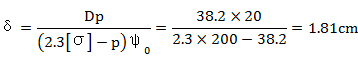81cm ＜ 2. 5cm (actual wall thickness) with enough strength.

Theoretical calculation wall thickness of zfs5100 column middle cylinder: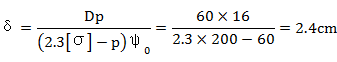The strength of 2.4cm ＞ 1.5cm (actual wall thickness) is not enough. The theoretical wall thickness of outer cylinder and middle cylinder of zz56k column can also be calculated

δ Outside = 1.7 (CM) < 1.95 (CM), the strength is enough.

δ Medium = 2.39 (CM) > 2.0 (CM), the strength is not enough.

It can be seen from the calculation that the wall thickness of the outer cylinder of the column is relatively abundant, and the proportion of expanding cylinder is relatively small} the wall thickness of the middle cylinder is relatively thin, and the proportion of expanding cylinder is relatively large. In other words, in the design process of the outer cylinder, the safety factor is n = 5 (in line with the requirements of the design manual n ≥ 5), while the safety factor of the middle cylinder is n = 3.3 ~ 4. This is one of the main reasons for the cylinder expansion of the column cylinder sometimes, and the calculation result is consistent with the actual situation.

② In the process of column design and checking calculation, the designers generally consider the column as hinged at both ends, no eccentric load, and the slender rod with equal cross-section is subject to axial load. Here, the influence of the clearance between the movable column and the small guide sleeve is omitted, and the movable column and the intermediate cylinder are regarded as one body, and their stability is studied by longitudinal pressure.

a. The calculation of the ultimate longitudinal bending force PR without eccentric load.

Taking zfs5100 column as the research object, the flexibility is calculated λ：

λ= ul/i (6-4)

Where u -- length coefficient of compression bar, hinged at both ends, u = 1;

Z -- the calculated length of piston rod, i.e. the distance between the connection point at the top of the movable column and the support point of the hydraulic cylinder when the movable column is fully extended, l = 335cm;

I-minimum inertia radius of cross section of movable column, I = = 3.5 (CM).

therefore

b. Look up the table λ 1 =100， λ 2 = 60 due to λ 2 < λ<λ Therefore, the critical stress is calculated by the linear formula.

σ c =a- σλ= ( 461 -2.568 × 95.7) × 102=215 × 102 (N/cm2)

Pc= σ cA - σ c（π/4）d2 =215 ×( 3.14/4) × 142 =3312kN

c. Calculation of safety factor.

u=Pc/P=3312/1200=2.75

Using the same method, we can calculate:

Zz560k column PC = 4944kn, u = 4.34;

Zzp5200 column PC = 5124kn, u = 3.9.

Through calculation, it can be seen that the margin of stability is not enough under this ideal stress state. In addition, the column is a combination, and there are gaps between piston and cylinder barrel, between movable column and guide sleeve, between intermediate cylinder and guide sleeve. Especially the appearance of transverse force, the movable column of column is affected by a bending stress as well as axial force. When the compressive and bending stresses exceed a certain value of the allowable stress, the movable column or intermediate cylinder will produce permanent bending deformation. When the flexible column or middle cylinder that produces bending deformation is in telescopic motion, it will produce mechanical scratch on its mating surface. At the same time, it also causes the outer cylinder and the middle cylinder to form an ellipse, resulting in the damage of the connecting thread and the failure of the sealing surface. The bending deformation of movable column and intermediate cylinder is shown in Figure P.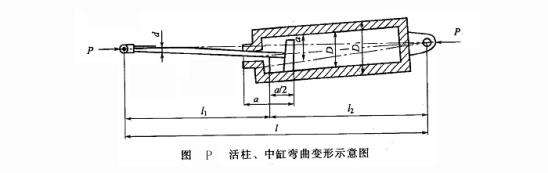③ The source of the transverse force on the column is V-shaped. The transverse force caused by the dead weight of the column is too small to be ignored. The left and right inclination of the support is the main source of the transverse force.

The base of the lower end of the column is hinged through two pins connected with the base. The left and right directions can be simplified as fixed connection (front beam facing the support). See the connection diagram Q and stress diagram R. the front and rear directions can be simplified as hinged connection. See Fig. s and U.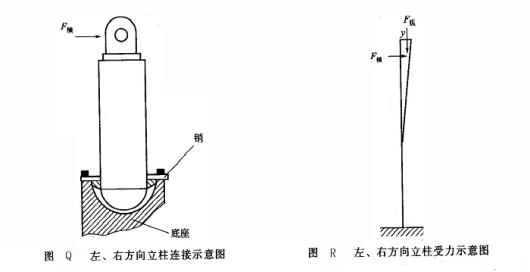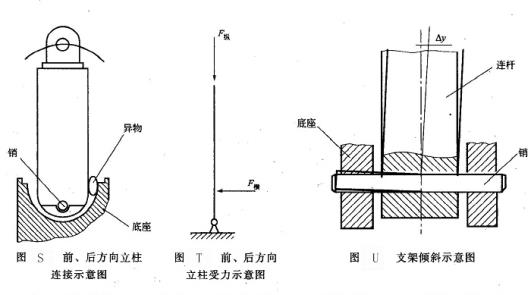Because of the complex geological conditions, the inclination of the working face and the unevenness of the roof and floor can force the support to incline; The base pin hole and four connecting rod pin hole of the bracket are elliptical and large, and the pin shaft is bent and refined, which also causes the bracket to tilt. The inclination of the support ay shall be equal to the deflection of the upper end of the column caused by a transverse force F (see Fig. U).

When the support tilts back and forth, there will be a certain amount of rotation of the column. Under normal circumstances, there will be no transverse force, but once the column nest enters into foreign matters, such as coal, gangue, metal, wood, etc., the transverse force will be generated (see Fig. s).

Home    Article    Failure analysis of hydraulic support of coal mine equipment_ Cause analysis of column damage